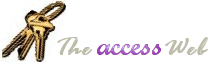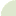Home  |   French  |   About  |   Search  | mvps.org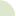What's New Table Of Contents Credits Netiquette 10 Commandments  Bugs Tables Queries Forms Reports Modules APIs Strings Date/Time General Downloads Resources Search Feedback mvps.org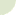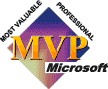Modules: Convert Currency (\$500) into words (Five Hundred Dollars) Author(s) Joe Foster(Q)    I'm looking to have Access convert the currency value "\$531.20" into the text "Five hundred thirty one and twenty cents." (A)    Use the following function provided by Joe Foster to convert the Currency into English words. ```'************ Code Start ********** 'This code was originally written by Joe Foster. 'It is not to be altered or distributed, 'except as part of an application. 'You are free to use it in any application, 'provided the copyright notice is left unchanged. ' 'Code Courtesy of 'Joe Foster ' ' Convert a currency value into an (American) English string Function English (ByVal N As Currency) As String Const Thousand = 1000@ Const Million = Thousand * Thousand Const Billion = Thousand * Million Const Trillion = Thousand * Billion If (N = 0@) Then English = "zero": Exit Function Dim Buf As String: If (N < 0@) Then Buf = "negative " Else Buf = "" Dim Frac As Currency: Frac = Abs(N - Fix(N)) If (N < 0@ Or Frac <> 0@) Then N = Abs(Fix(N)) Dim AtLeastOne As Integer: AtLeastOne = N >= 1 If (N >= Trillion) Then Debug.Print N Buf = Buf & EnglishDigitGroup(Int(N / Trillion)) & " trillion" N = N - Int(N / Trillion) * Trillion ' Mod overflows If (N >= 1@) Then Buf = Buf & " " End If If (N >= Billion) Then Debug.Print N Buf = Buf & EnglishDigitGroup(Int(N / Billion)) & " billion" N = N - Int(N / Billion) * Billion ' Mod still overflows If (N >= 1@) Then Buf = Buf & " " End If If (N >= Million) Then Debug.Print N Buf = Buf & EnglishDigitGroup(N \ Million) & " million" N = N Mod Million If (N >= 1@) Then Buf = Buf & " " End If If (N >= Thousand) Then Debug.Print N Buf = Buf & EnglishDigitGroup(N \ Thousand) & " thousand" N = N Mod Thousand If (N >= 1@) Then Buf = Buf & " " End If If (N >= 1@) Then Debug.Print N Buf = Buf & EnglishDigitGroup(N) End If If (Frac = 0@) Then Buf = Buf & " exactly" ElseIf (Int(Frac * 100@) = Frac * 100@) Then If AtLeastOne Then Buf = Buf & " and " Buf = Buf & Format\$(Frac * 100@, "00") & "/100" Else If AtLeastOne Then Buf = Buf & " and " Buf = Buf & Format\$(Frac * 10000@, "0000") & "/10000" End If English = Buf End Function ' Support function to be used only by English() Private Function EnglishDigitGroup (ByVal N As Integer) As String Const Hundred = " hundred" Const One = "one" Const Two = "two" Const Three = "three" Const Four = "four" Const Five = "five" Const Six = "six" Const Seven = "seven" Const Eight = "eight" Const Nine = "nine" Dim Buf As String: Buf = "" Dim Flag As Integer: Flag = False 'Do hundreds Select Case (N \ 100) Case 0: Buf = "": Flag = False Case 1: Buf = One & Hundred: Flag = True Case 2: Buf = Two & Hundred: Flag = True Case 3: Buf = Three & Hundred: Flag = True Case 4: Buf = Four & Hundred: Flag = True Case 5: Buf = Five & Hundred: Flag = True Case 6: Buf = Six & Hundred: Flag = True Case 7: Buf = Seven & Hundred: Flag = True Case 8: Buf = Eight & Hundred: Flag = True Case 9: Buf = Nine & Hundred: Flag = True End Select If (Flag <> False) Then N = N Mod 100 If (N > 0) Then If (Flag <> False) Then Buf = Buf & " " Else EnglishDigitGroup = Buf Exit Function End If 'Do tens (except teens) Select Case (N \ 10) Case 0, 1: Flag = False Case 2: Buf = Buf & "twenty": Flag = True Case 3: Buf = Buf & "thirty": Flag = True Case 4: Buf = Buf & "forty": Flag = True Case 5: Buf = Buf & "fifty": Flag = True Case 6: Buf = Buf & "sixty": Flag = True Case 7: Buf = Buf & "seventy": Flag = True Case 8: Buf = Buf & "eighty": Flag = True Case 9: Buf = Buf & "ninety": Flag = True End Select If (Flag <> False) Then N = N Mod 10 If (N > 0) Then If (Flag <> False) Then Buf = Buf & "-" Else EnglishDigitGroup = Buf Exit Function End If 'Do ones and teens Select Case (N) Case 0: ' do nothing Case 1: Buf = Buf & One Case 2: Buf = Buf & Two Case 3: Buf = Buf & Three Case 4: Buf = Buf & Four Case 5: Buf = Buf & Five Case 6: Buf = Buf & Six Case 7: Buf = Buf & Seven Case 8: Buf = Buf & Eight Case 9: Buf = Buf & Nine Case 10: Buf = Buf & "ten" Case 11: Buf = Buf & "eleven" Case 12: Buf = Buf & "twelve" Case 13: Buf = Buf & "thirteen" Case 14: Buf = Buf & "fourteen" Case 15: Buf = Buf & "fifteen" Case 16: Buf = Buf & "sixteen" Case 17: Buf = Buf & "seventeen" Case 18: Buf = Buf & "eighteen" Case 19: Buf = Buf & "nineteen" End Select EnglishDigitGroup = Buf End Function '************ Code End **********```© 1998-2010, Dev Ashish & Arvin Meyer, All rights reserved. Optimized for Microsoft Internet Explorer